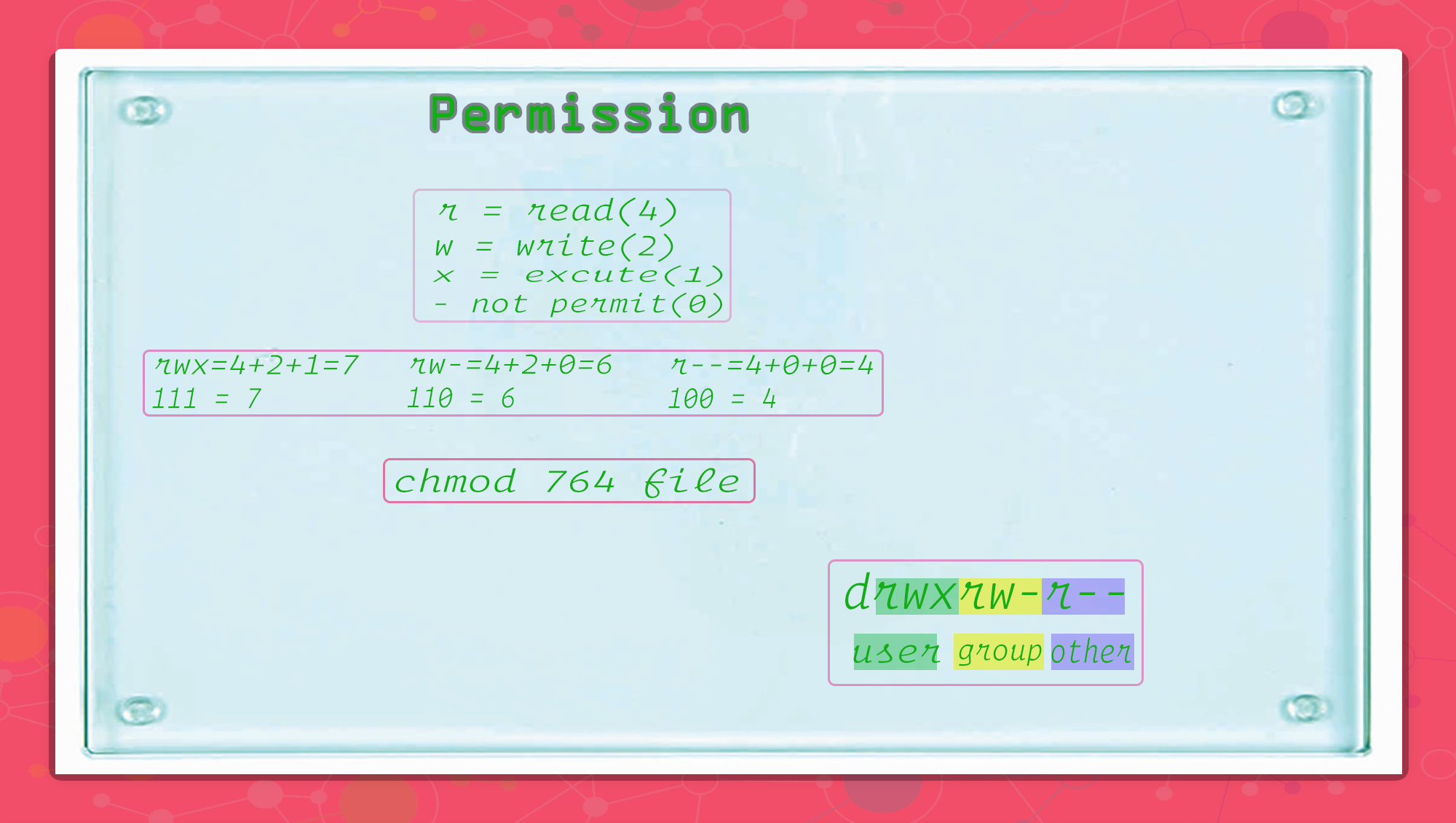# chmod permission

## what is chmod

chmod means `change mode` . chmod can change the file permission.

short hand Full
u user
g group
o other

## chmod basic

``````# cli
ls -l
# output
drwxrw-r--
``````

user = first 3 position without d = rwx group = next 3 position = rw- other = last 3 position = r–

• first position `d` is `type`

## permission listscli name Full name number value
w write 2
x excute 1
- not permit 0
``````user = rwx => 4+2+1 = 7
group = rw- => 4+2+0 = 6
others = r-- => 4+0+0 = 4

last result => chmod 764 => rwxrw-r--
``````

### binary system

• permission = 1
• no permit = 0
``````user = rwx => 111 => binary to decimal => 7
group = rw- => 110 => binary to decimal => 6
others = r-- => 100 => binary to decimal => 4

last result=> chmod 764 => rwxrw-r--
``````

## chmod pettern

`chmod <options> <permissions> <filename>`

• options
• `-R` recursive, i.e. include objects in subdirectories
• `-f` force, forge ahead with all objects even if errors occur
• `-v` verbose, show objects processed

## how to use chmod

``````chmode u+rwx, g+rw-, o+r-- desired_file
# number system
chmod 764 desired_file
``````